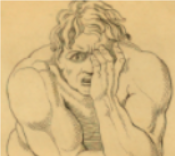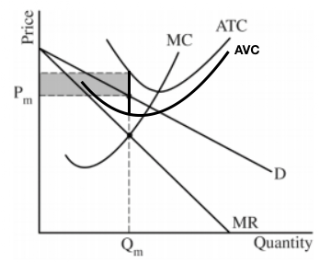## Saturday, July 7, 2018

### 2018 AP Microeconomics FRQ #3

2018 AP Microeconomics FRQ #3
(a) Nirali spends 3 hours studying microeconomics and 2 hours studying history. Calculate her gain from the second hour spent studying history.

First, we should recognize that the Expected Score is the expected Output (grade) gained by studying a certain number of hours. For instance, if I study one hour my expected score will be a 40 on the exam, and if I study an additional hour (2) I'll add 20 points to my score. My marginal score from 1 hour studied to 2 hours studied is 20. I gained 20 points by studying and extra hour.

(b) Calculate Nirali's opportunity cost of time spent studying that second hour of history.

(c) Assume that Narali increases the time she allocates to studying history. What happens to the opportunity cost of studying history? Explain.

We would assume that opportunity cost would increase. Her Opportunity cost (what she gives up) by studying more history is a worse score in Micro.

(d) Assume that Narali has a goal of maximizing the sum of her test scores. How many hours should she study for each?

It's clear by looking at the marginal gains, that the best choice is 3 hours of history and 2 hours of Micro.
At 3 hours of history and 2 of micro we gain a total score (utility) of 72 + 82 = 154
At 2 hours of history and 3 of micro we gain a total score (utility) of 60 + 90 = 140
At 4 hours of history and 1 of micro we gain a total score (utility) of 77 + 60 = 137

(e) Nirali gains an additional hour where will she allocate it? Explain using marginal analysis.

The 3rd hour of micro gains her 8 points while the 4th hour of history only gains her 5 points.
8 > 5 so choose the additional hour of micro.

### 2018 AP Microeconomics FRQ #2

2018 AP Microeconomics FRQ #2

(a) Identify the type of market failure illustrated by the graph. Explain.
The type of market failure is an Positive Consumption "Externality".

(b) Using the numbers on the graph, identify the market equilibrium price and quantity.

(c) Using the labeling on the graph, identify the area representing DWL at the quantity identified in part (b).
Part (b) is the equilibrium price of \$6 and quantity of 16, the DWL at that quantity is EDF.

(d) Suppose the government is granting a subsidy to correct the market failure. What is the dollar value of the per unit subsidy to achieve the SOQ (Socially Optimal Quantity)?

(e) Suppose the government installs a price floor at \$8,
(i) How many units will producers and consumers exchange? (8)
(ii) Does the Price floor correct the market failure?
NO, it actually makes it worse,, society would like 24 units consumed but after the price floor only 8 units are consumed.College Board Thou canst not vex me with inconsistent mind, Since that my life on thy revolt doth lie.O, what a happy externality do I find,Happy to have thy love, happy to die!

### 2018 AP Microeconomics FRQ #1

2018 AP Microeconomics FRQ #1

(A) Draw a correctly labeled graph for Single Cinema, and show
(i) The profit maximizing price and quantity of tickets labeled as Pm and Qm respectively.
(ii) The area representing the negative economic profit (loss) shaded completely
(B) Explain why Single continues to operate in the short-run despite earning negative profits.

Understand that as long as Single is at least paying some of its fixed costs and it isn't at the shut-down point (P = min AVC) then it should continue to operate.The distance between the AVC and the ATC represents fixed costs (costs that don't change with additional output) if by being in business we are making a loss but are still paying some of our fixed costs we should remain in business because if we shut down, all of our fixed costs are due.
Think of it like this, we pay \$500 a month in rent, rent that is due every month no matter if we produce any product or not. If by being open we are making a loss of \$100 a month, we are still paying \$400 of our fixed costs (rent). If we shut down we owe the entire \$500. As long as we stay open even when making a loss we will be covering some of our fixed costs. If the price falls below the minimum of the AVC (Shut-down point) we can't cover any of our fixed costs and now some of our VC (variable costs) can't be paid, variable costs include labor.

(C) Would Single's total revenue increase, decrease or stay the same if it decides to sell one ticket less than Qm? Explain.

Recognize that if Single reduces it's quantity by 1 the price will increase, as the monopolist only produces in the elastic section of its demand curve, an increase in price means a decrease in total revenue.

(D) Single hires workers in a perfectly competitive labor market with a downward sloping demand curve. Suppose the number of workers available in the market decreases.
(i) What will happen to the wage rate? Explain.
(ii) What will happen to the MRP of the last worker hired.  Explain.

(i) Number of workers decreases = Supply of labor decreases = Wage increases
(ii) Understand that the MRP = MP x Pg (Marginal Product x the Price of the Good Sold)
The MP of each worker decreases as more workers are added to the fixed capital = diminishing marginal returns so the reverse of this would be that every person not hired (fired) would have a hire MRP that the preceding one.

## Friday, July 6, 2018

### 2018 Macroeconomics FRQ #2

2018 Macroeconomics FRQ #2

2. Assume the economy of Ucheleand is currently at full employment. The government of Ucheland reduces the tax rate on household interest earnings.

1st you must know what household interest earnings are -   interest that people earn on the savings in their bank accounts. If the government reduces the tax on those savings, then people will save more...

(a) What will happen to private savings in Ucheland?
Private savings will increase.

(b) Draw a CLG of the loanable funds market, and show, the effect of the change in private savings identified in (a) on the equilibrium real interest rate.
(c) Given the Real Interest Rate change identified in part (b), answer the following.

(i) What is the short-run effects on aggregate demand? Explain.

If the supply of loanable funds increases, driving the RIR to fall, then citizens will be more likely to take out loans and invest. Investing will shift the AD curve rightward.

(ii) What is the long run effect on the potential real GDP. Explain.
I believe this question is asking about growth in the Long-Run.
Potential GDP is the curve on the PPC. = Any point on the PPC boundary is where resources are Allocatively efficient and referred to as Potential GDP. Potential, as this is the best a society could achieve with all available resources actively engaged.

PPC Cheat Sheet here

If there is more investment, then we assume that some of this new investment will be investment in capital formation, this new capital creation will push out the PPC boundary = more LR Growth.

### 2018 AP Macroeconomics FRQ#1

2018 AP Macroeconomics FRQ#1
1) Assume the US economy is in recession.

(a) Draw a CLG of the LRAS, SRAS, and AD curves, Show each of the following.
(i) Current price level, labeled PL1
(ii) Current output, labeled Y1US in Recession

2) Now assume the Euro-Zone, a major trading partner of the US, enters into a recession.

(i) What will be the effect on US exports to the Euro-Zone? Explain.

The effects of a recession in the Euro-Zone will lead to less US goods being purchased by Euro-Zone citizens. Less purchase of goods from the US means that the US exports will decline. You could also talk about prices of goods will fall during a recession in the Euro Zone and therefore Euro citizens will find their own goods relatively cheaper or that aggregate incomes in the Euro-zone will fall and that will make the US produced goods relatively more expensive therefore less will be purchased from the US. If US exports decrease then AD shifts left.

(ii) On your graph and part (a), show, the effect of the change identified in part (b)(i) on real output in the US.

(iii) What will be the change in identified in part (b)(ii) on unemployment in the US.
If countries don't buy our stuff (exports fall) then AD will decrease, the US slides further into a recession and a recession is characterized by a lower PL, less output, and higher levels of unemployment.

3) Assume the Euro-Zone recession causes a decrease in demand for US dollars in the FOREX.
(i) Will the euro appreciate, depreciate, or remain unchanged against the US dollar? Explain.

If the Euro-Zone isn't demanding our goods then the demand for US dollars is decreasing. If the demand for US dollars is falling then the value of the US dollar must be depreciating. That means that the Euro must be appreciating relative to the US dollar.

FOREX cheat sheet here

(ii) Draw a CLG of the FOREX for \$ dollars, and show the decrease in demand for dollars on the exchange rate for dollars.
I read this two ways, which in essence are saying the same thing
1) the demand for US dollars is decreasing therefore the value of the US dollar is decreasing and/or
2) the number of Euro's it takes to buy 1 US dollar is decreasing, meaning that the Euro's value must be appreciating.

D) Assume the US implements expansionary monetary and fiscal policies. In the absence of complete crowding out, what will be the effect of these policies on each of the following?

(i) Aggregate Demand in the US - Aggregate Demand will increase because of the expansionary monetary and fiscal policies.

(ii) Price Level - The price level will increase, as AD shifts rightward due to the expansionary policies the PL will increase.

(iii) Interest Rates in the US  - Understand that as the US Government uses expansionary fiscal policy (GS increases or Taxes decreased) the (RIR) Real Interest Rate will increase and as the FED uses expansionary monetary policy (Buys Bonds, decreases the (RRR) Required Reserve Ratio, or the (DR) Discount Rate) the Nominal Interest Rate will be increased as the (MS) Money Supply increases.

Monetary & Fiscal Combo effects cheat sheet here.

### Balance of Payments

If your pushed for time, remember this.

Remember
If there is a Surplus in the Current Account then there is a Deficit in the Capital Account.
&
If there is a Deficit in the Current Account then there is a Surplus in the Capital Account.